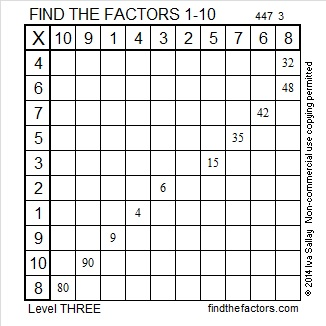# 305 and Level 3

• 305 is a composite number.
• Prime factorization: 305 = 5 x 61
• The exponents in the prime factorization are 1 and 1. Adding one to each and multiplying we get (1 + 1)(1 + 1) = 2 x 2 = 4. Therefore 305 has exactly 4 factors.
• Factors of 305: 1, 5, 61, 305
• Factor pairs: 305 = 1 x 305 or 5 x 61
• 305 has no square factors that allow its square root to be simplified. √305 ≈ 17.464Print the puzzles or type the factors on this excel file: 10 Factors 2014-11-24

A Logical Approach to FIND THE FACTORS: Find the column or row with two clues and find their common factor. Write the corresponding factors in the factor column (1st column) and factor row (top row).  Because this is a level three puzzle, you have now written a factor at the top of the factor column. Continue to work from the top of the factor column to the bottom, finding factors and filling in the factor column and the factor row one cell at a time as you go.